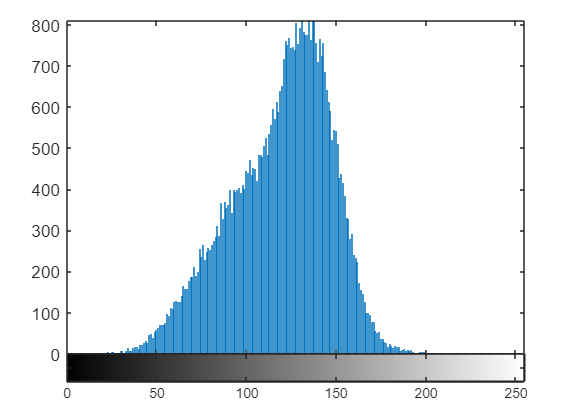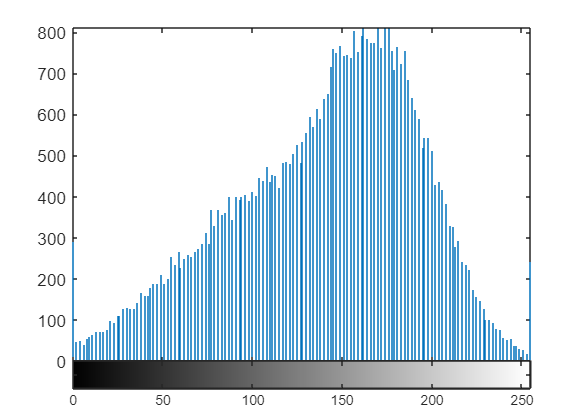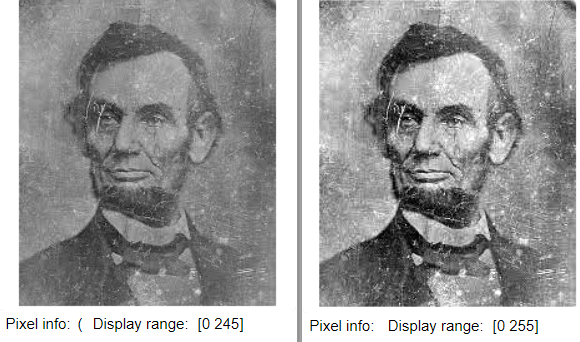# How to Normalize a Histogram in MATLAB?

Histogram normalization is a technique to distribute the frequencies of the histogram over a wider range than the current range. This technique is used in image processing too. There we do histogram normalization for enhancing the contrast of poor contrasted images.

Formula:Here ./ and .* means operation has to be performed element-wise.

Steps:

• Convert color image into grayscale.
• Display histogram.
• Observe maximum and minimum intensities from the histogram.
• Change image type from uint8 to double.
• Apply a formula for histogram normalization.
• Convert back into unit format.
• Display image and modified histogram.

Example:

## Matlab

 `% MATLAB code for ` `% Histogram normalisation.` `% Read the image.` `k=imread(``"lincoln.jfif"``);`   `% Convert into grayscale` `k1=rgb2gray(k);`   `% Display the image and histogram.` `imtool(k1,[]);` `imhist(k1);`   `% Set the minimum and maximum` `% Values from histogram.` `min=45;` `max=180;`   `% Convert image into double.` `k2=double(k1);`   `% Apply the formula.` `k3=(k2-min)./(max-min);`   `% Multiply with maximum possible value.` `k4=k3.*255;`   `% Convert the final result into uint8.` `k5=uint8(k4);`   `% Display the enhanced image and histogram.` `imtool(k5,[]);` `imhist(k5);`

Output:Figure: Original histogramFigure: Normalized histogramFigure: Images before and after normalization

Code Explanation: